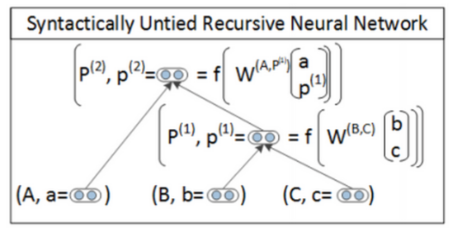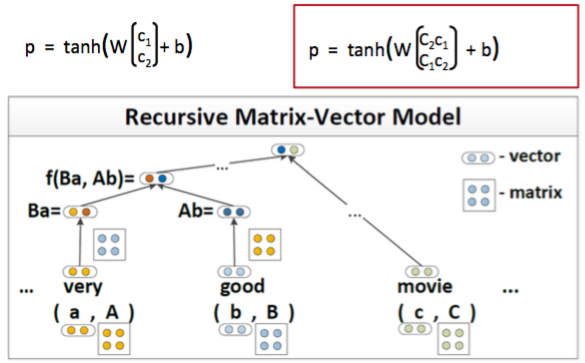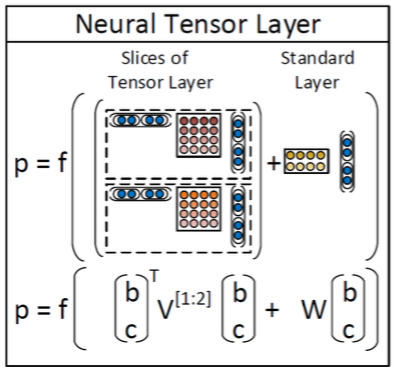# CS224d笔记5——递归神经网络（Recusive Neural Network, RNN）

RNN适用于有嵌套层次和内在递归结构的任务。目前RNNNLP领域的应用主要有句法分析和 句子表示。假设一个句子的含义是由句子中词的含义和词的组合方式决定的 ，word2vec已经一定程度上说明可以用向量来表示词的含义，词的组合规则从句法的角度 来看可以理解成句法树，我们可以通过遍历句法树来构建RNN（递归的时候使用同一组参数 ）来生成句子的表示。这样生成句子、短语的表示考虑了词的顺序、词的组合和词的含义。 其实我们可以利用RNN来同时学习句子的句法结构和句子的向量表示。

RNN用于结构预测的时候需要用到一个max-margin目标函数，暂时没有看懂这个目标函数。 所以本文仅介绍在有一个句法树的前提下来生成句子表示的过程，同时结合一个简单的情感 分析任务来解释RNN的前向传播和反向传播过程。

## 单层RNN建模情感分析

$$h^{(1)}=\tanh(W^{(1)} \begin{bmatrix} L_{29}\\ L_{430} \end{bmatrix} + b^{(1)})$$

$$h^{(1)}=\tanh(W^{(1)} \begin{bmatrix} h_{left}^{(1)}\\ h_{right}^{(1)} \end{bmatrix} + b^{(1)})$$
$$\hat{y}=\text{softmax}(Uh^{(1)}+b^{(s)})$$

$$h_{left}^{(1)}$$是左孩子的输出向量，$$h_{left}^{(1)}$$是右孩子的输出向量 （这两个向量都可能是词向量）。最后经过一个softmax得到整个句子的分类，在情感分类任务中 $$U\in \mathbb{R}^{5\times d}$$$$b^{(s)}\in\mathbb{R}^5$$

1. 得到左孩子的表示
2. 得到右孩子的表示
3. 得到根节点的表示

## 后向传播

1. 计算根节点的误差
2. 计算左孩子的误差
3. 计算右孩子的误差

$$\text{CE}(y,\hat{y})=-\sum_i y_i\log(\hat{y}_i)$$

## RNN改进

### Syntactically Untied SU-RNN

SU-RNN的主要思想是用PCFG先给句法树种的每个节点和词打上标签。 然后DT-NP拼接的时候用一种$$W$$VP-NP拼接的时候用另一个$$W$$，这样可以提高模型的描述能力。SU-RNN比之前的同一个$$W$$的模型有了一定的改进，但是还解决不了修饰词的问题，例如very 这个词会本身就会使得另一个词的词意变强，通过SU-RNN的这种线性插值的方法无法让某个向量 去放大另一个向量。界面介绍两个可以描述这类问题的模型MV-RNNRNTN

### MV-RNN’s (Matrix-Vector Recursive Neural Networks)

MV-RNN中每个词不仅有一个词向量，还有一个矩阵，例如下图中的词very有一个向量a 还有一个矩阵A。当verygood组合的时候首先需要把当前词的向量和另一个词的矩阵相乘， 例如very的向量a需要和good的矩阵B相乘，然后再通过普通RNN的计算公式得到新节点的 隐藏层表示。very这个词的矩阵可以初始化成单位矩阵乘上某个正数，这样和对方的向量相乘 的时候就可以放大对方的向量。### RNTNs (Recursive Neural Tensor Network)

RNTN首先对左右孩子的$$h^(1)$$进行拼接得到$$x\in\mathbb{R}^{2d}$$，在普通的RNN中也这么做 但是普通的RNN直接把$$x$$进行线性变化之后就经过非线性神经单元，而在RNTN中首先对$$x$$进行 一次二次变换，然后加上线性变换，最后通过非线性单元：

$$h^{(1)}=\tanh(x^TVx + W^{(1)}x)$$